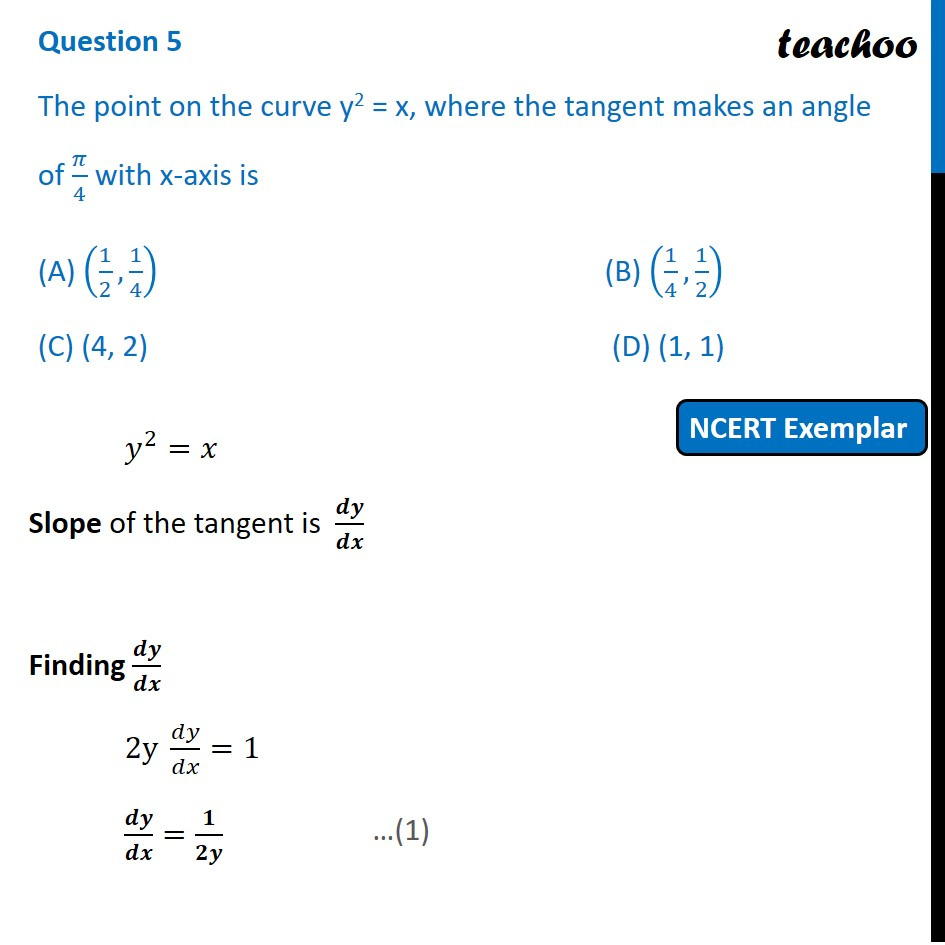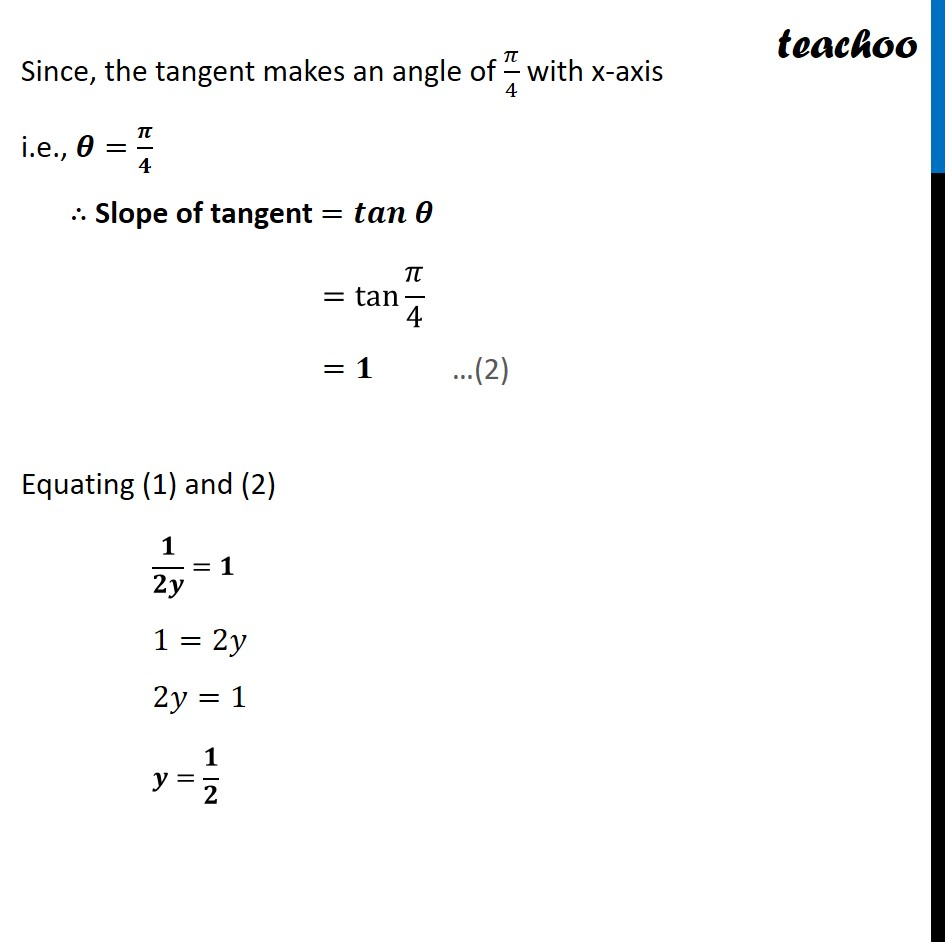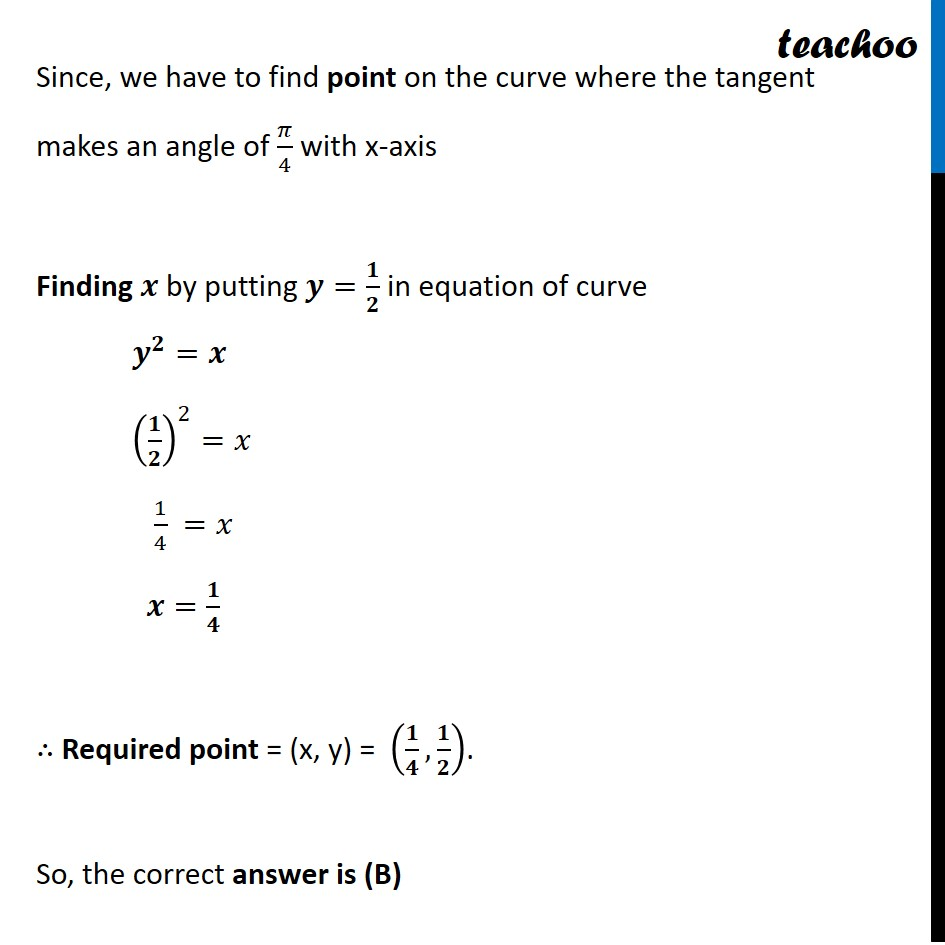NCERT Exemplar - MCQs

Chapter 6 Class 12 Application of Derivatives
Serial order wise

## (C) (4, 2)                   (D) (1, 1)

This question is similar to Example 17 - Chapter 6 Class 12 - Application of DerivativesLearn in your speed, with individual attention - Teachoo Maths 1-on-1 Class

### Transcript

Question 5 The point on the curve y2 = x, where the tangent makes an angle of 𝜋/4 with x-axis is (A) (1/2, 1/4) (B) (1/4, 1/2) (C) (4, 2) (D) (1, 1) 𝑦^2=𝑥 Slope of the tangent is 𝒅𝒚/𝒅𝒙 Finding 𝒅𝒚/𝒅𝒙 2y 𝑑𝑦/𝑑𝑥=1 𝒅𝒚/𝒅𝒙=𝟏/𝟐𝒚 Since, the tangent makes an angle of 𝜋/4 with x-axis i.e., 𝜽=𝝅/𝟒 ∴ Slope of tangent =𝒕𝒂𝒏 𝜽 =tan⁡〖𝜋/4〗 =𝟏 Equating (1) and (2) 𝟏/𝟐𝒚=𝟏 1=2𝑦 2𝑦=1 𝒚=𝟏/𝟐 Since, we have to find point on the curve where the tangent makes an angle of 𝜋/4 with x-axis Finding 𝒙 by putting 𝒚=𝟏/𝟐 in equation of curve 𝒚^𝟐=𝒙 (𝟏/𝟐)^2=𝑥 1/4 =𝑥 𝒙=𝟏/𝟒 ∴ Required point = (x, y) = (𝟏/𝟒,𝟏/𝟐). So, the correct answer is (B)